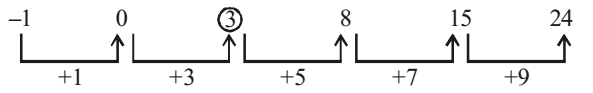Home » Verbal Reasoning » Number Series » Question

#### Number Series

Direction: What will come in the place of question mark (?) in the following number.

1. –1, 0, ?, 8, 15, 24
1. 4
2. 3
3. 2
4. 1
5. None of these
##### Correct Option: B

The number pattern is described in the below image.
As per figure we can see the number series is in specific pattern which will help us to find the next number.
It is clear that we are getting the next terms on adding + 1 , + 3 , + 5 , + 7 , + 9 in previous term respectively.
First term = - 1
Second term = First term + 1
Second term = -1 + 1 = 0
Third term = Second term + 3
Third term = 0 + 3 = ( ? )
∴ ( ? ) = 3
Similarly we can calculate the all others terms
Fourth term = 8
Fifth term= 15